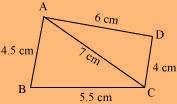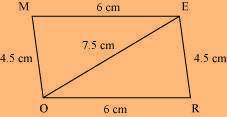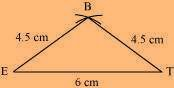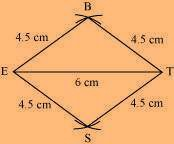# Practical Geometry Chapter 4 Class 8 Maths NCERT Textbook With Solutions PDF

NCERT Solutions for Class 8 Maths Chapter 4′ PDF Quick download link is given at the bottom of this article. You can see the PDF demo, size of the PDF, page numbers, and direct download Free PDF of ‘Ncert Class 8 Maths Chapter 4 Exercise Solution’ using the download button.

### Chapter 4:Practical Geometry

#### 4.1 Introduction

You have learned how to draw triangles in Class VII. We require three measurements (of sides and angles) to draw a unique triangle.

Since three measurements were enough to draw a triangle, a natural question arises whether four measurements would be sufficient to draw a unique four-sided closed figure, namely, a quadrilateral.

We shall learn how to construct a unique quadrilateral given the following measurements:
• When four sides and one diagonal are given.
• When two diagonals and three sides are given.
• When two adjacent sides and three angles are given.
• When three sides and two included angles are given.
• When other special properties are known.

#### 4.2.2 When two diagonals and three sides are given

When four sides and a diagonal were given, we first drew a triangle with the available data and then tried to locate the fourth point. The same technique is used here.

#### 4.2.4 When three sides and two included angles are given

Under this type, when you draw a rough sketch, note carefully the “included” angles in particular.

### NCERT Solutions Class 8 Maths Chapter 4 Practical Geometry

(i) Quadrilateral ABCD AB = 4.5 cm

BC = 5.5 cm

CD = 4 cm AD = 6 cm AC = 7 cm

Solution:The rough sketch of the quadrilateral ABCD can be drawn as follows.

(1) ∆ABC can be constructed by using the given measurements as follows.

(2) Vertex D is 6 cm away from vertex A. Therefore, while taking A as centre, draw an arc of radius 6 cm.

(3) Taking C as the centre, draw an arc of radius 4 cm, cutting the previous arc at point D. Joint D to A and C.

(ii) Quadrilateral JUMP JU = 3.5 cm

UM = 4 cm MP = 5 cm PJ = 4.5 cm PU = 6.5 cm

Solution:

The rough sketch of the quadrilateral JUMP can be drawn as follows.

(1) ∆ JUP can be constructed by using the given measurements as follows.

(2) Vertex M is 5 cm away from vertex P and 4 cm away from vertex U. Taking P and U as centres, draw arcs of radii 5 cm and 4 cm respectively. Let the point of intersection be M.

(3) Join M to P and U.

(iii) Parallelogram MORE

OR = 6 cm

Solution:

RE = 4.5 cm

EO = 7.5

We know that opposite sides of a parallelogram are equal in length and also these are parallel to each other.

i.e., ME = OR, MO = ERThe rough sketch of the parallelogram MORE can be drawn as follows.

(1) ∆ EOR can be constructed by using the given measurements as follows.

(2) Vertex M is 4.5 cm away from vertex O and 6 cm away from vertex E. Therefore, while taking O and E as centres, draw arcs of 4.5 cm radius and 6 cm radius respectively. These will intersect each other at point M.

(3) Join M to O and E.

MORE is the required parallelogram.

(iv) Rhombus BEST

BE = 4.5 cm

ET = 6 cm

Solution:

We know that all sides of a rhombus are of the same measure. Hence, BE = ES = ST = TB The rough sketch of the rhombus BEST can be drawn as follows.(1) ∆ BET can be constructed by using the given measurements as follows.

(2) Vertex S is 4.5 cm away from vertex E and also from vertex T. Therefore, while taking E and T as centres, draw arcs of 4.5 cm radius, which will be intersecting each other at point S.

(3) Join S to E and T.

NCERT Solution For Class 8 Maths Chapter 4 ImageBEST is the required rhombus.